# RegEx - Validate Phone Numbers

## RegEx - Validate Phone Numbers

• Now a regex pattern is built step by step to check out the ten-digit phone number with the pattern 041 344-4567
 Number Pattern Explanation \b To define a starting boundary for the number. 041 \d{3} To define presence of three digits. \s To define a space. 344 \d{3} Again to define three digits. - - To define hyphen. 4567 \d{4} To define four digits. \$ To define an ending boundary for the number.
• Hence finally the regex pattern matching the string “041 344-4567” should be \b\d{3}\s\d{3}-\d{4}\$. Below follows the sample code to implement the pattern check with C#.

## Sample code :

`using System;using System.Collections.Generic;using System.Linq;using System.Text;using System.Threading.Tasks;namespace Regex_FirstExample{class Program{    static void Main(string[] args)    {        string number = "041 567-9876";   // number to check        string pattern = @"\b\d{3}\s\d{3}-\d{4}\$"; // pattern to  check        System.Console.WriteLine("The number is : " + number);        System.Console.WriteLine("The pattern string is : " + pattern);        if (System.Text.RegularExpressions.Regex.IsMatch(number, pattern))        // regex to validate the number        {             System.Console.WriteLine(" The given number is a valid pattern");        }        else        {             System.Console.WriteLine(" The given number is an invalid pattern");        }        // Keep the console window open in debug mode.         System.Console.WriteLine("Press any key to exit.");        System.Console.ReadKey()    }}}`

## Code Explanation :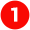The libraries are imported by default in C# class file.

`using System;using System.Collections.Generic;using System.Linq;using System.Text;using System.Threading.Tasks;`

• Here System is the parent library for C# code. Collections.Generic allow to generate strongly typed collections for better performance.
• The System.Text namespace is a holder for classes to represent ASCII and Unicode character encodings. RegularExpressions class is a child class of System.Text namespace.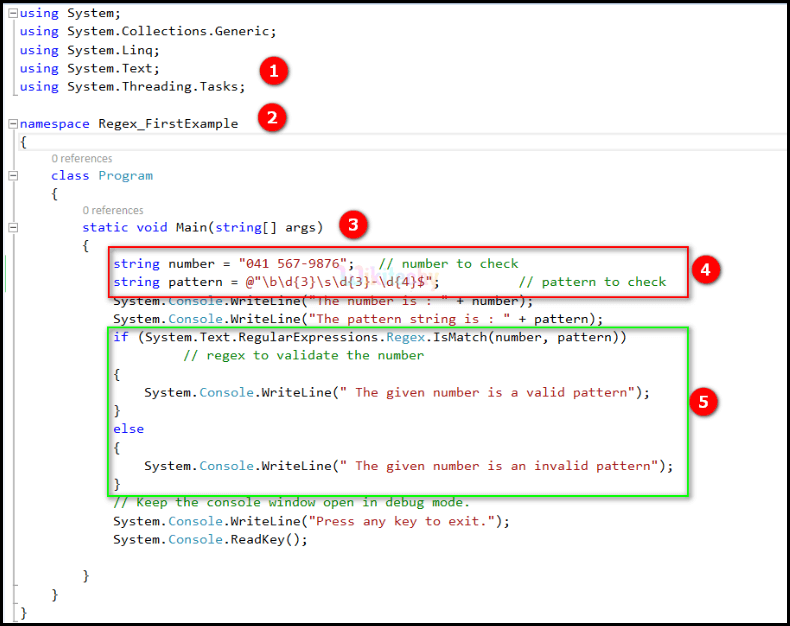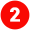The code namespace Regex_FirstExample defines a holder to contain one or more class. Here it contains a class named “Program”.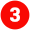The line of code static void Main(string[] args) defines a static  method called Main, whose elements can be called without an instance. It contains a string array as parameter, to receive input from the console window.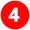The lines of code below declare two string variables, one with a string of numbers and other with a string pattern to match a number pattern as follows:

`string number = "041 567-9876"; // number to checkstring pattern = @"\b\d{3}\s\d{3}-\d{4}\$"; // pattern to check`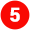Now the pattern string is checked against the string of numbers and if the check satisfies, a message is shown at the console as “The given number is a valid pattern” else “The given number is an invalid pattern”. This is done by the following lines of code:

`if (System.Text.RegularExpressions.Regex.IsMatch(number, pattern))// regex to validate the number{    System.Console.WriteLine(" The given number is a valid pattern");}else{    System.Console.WriteLine(" The given number is an invalid pattern");}`

## Output :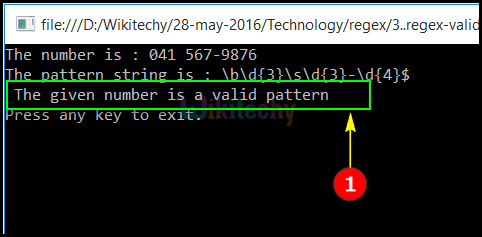The string pattern "\b\d{3}\s\d{3}-\d{4}\$" checks whether the string "041 567-9876" contains the same pattern. Since the check is satisfied here, the output is displayed as “The given number is a valid pattern”.

Try out with different number as follows:

`string number = "041 5678-9876";   // number to checkstring pattern = @"\b\d{3}\s\d{3}-\d{4}\$";  `
• The output is => The given number is an invalid pattern

Try out with different number as follows:

`string number = "941 567-9876";   // number to checkstring pattern = @"\b\d{3}\s\d{3}-\d{4}\$";  `
• The output is => The given number is a valid pattern.
• But the number should start with “0” and it’s not “9”. So it indicates that additional validation is required to set “0” as the first letter. The next sample code depicts this pattern.
• Step by step pattern build for ten-digit phone number with the pattern of 041 344-4567.
 Number Pattern Explanation 0 ^0 To define the starting number as 0. 41 \d{2} To define presence of two digits. \s To define a space. 344 \d{3} Again to define three digits. - - To define hyphen. 4567 \d{4} To define four digits. \$ To define an ending boundary for the number.
• Hence the exact regex pattern matching the required string should be as "^0\d{2}\s\d{3}-\d{4}\$".

Note:

• @ symbol used in the programs as an escape sequence detector. \d won’t be clearly understood by the compiler, if it is placed inside double quotes. Hence this can be cleared by adding @ symbol before “”.

## Code Explanation :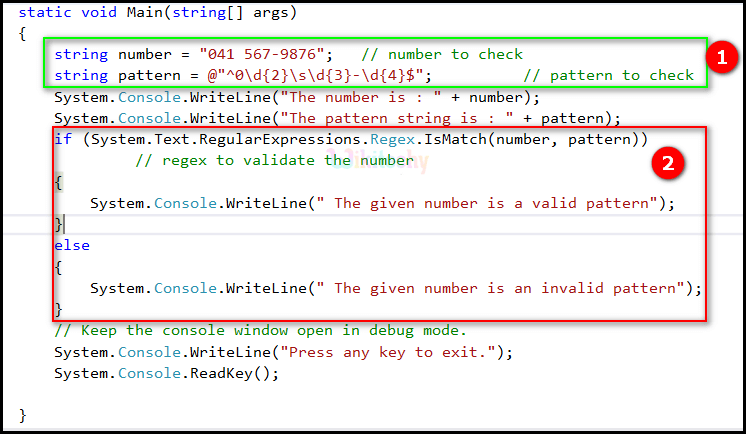The lines of code below declare two string variables, one with a string of numbers and other with a string pattern to match a number pattern as follows:

`string number = "041 567-9876"; // number to checkstring pattern = @"^0\d{2}\s\d{3}-\d{4}\$";     // pattern to check`Now the pattern string is checked against the string of numbers and if the check satisfies, a message is shown at the console as “The given number is a valid pattern” else “The given number is an invalid pattern”. This is done by the following lines of code:

`if (System.Text.RegularExpressions.Regex.IsMatch(number, pattern))// regex to validate the number{System.Console.WriteLine(" The given number is a valid pattern");}else{	System.Console.WriteLine(" The given number is an invalid pattern");}`

## Output :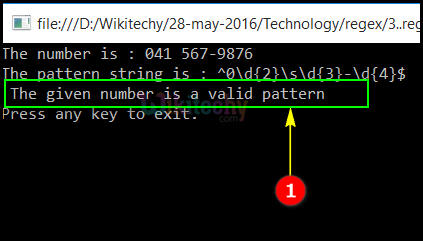The string pattern “^0\d{2}\s\d{3}-\d{4}\$" checks whether the string "041 567-9876" contains the same pattern. Since the check is satisfied here, the output is displayed as “The given number is a valid pattern”.

Another Pattern Variation :

• The number should start with 04 and if it has 03 then it should be an invalid pattern. Below follows the pattern build strategy for the number: 041 567-9876
 Number Pattern Explanation 04 ^04 To define the starting number as 04. 1 \d{1} To define presence of single digit. \s To define a space. 344 \d{3} Again to define three digits. - - To define hyphen. 4567 \d{4} To define four digits. \$ To define an ending boundary for the number.
• The regex pattern string should be as "^04\d{1}\s\d{3}-\d{4}\$";

## Sample code :

`using System;using System.Collections.Generic;using System.Linq;using System.Text;using System.Threading.Tasks;namespace Regex_FirstExample{class Program{static void Main(string[] args){string number = "041 567-9876";   // number to checkstring pattern = @"^04\d{1}\s\d{3}-\d{4}\$";    // pattern to checkSystem.Console.WriteLine("The number is : " + number);System.Console.WriteLine("The pattern string is : " + pattern);if (System.Text.RegularExpressions.Regex.IsMatch(number, pattern))// regex to validate the number{System.Console.WriteLine(" The given number is a valid pattern");}else{System.Console.WriteLine(" The given number is an invalid pattern");}// Keep the console window open in debug mode.System.Console.WriteLine("Press any key to exit.");System.Console.ReadKey();}}}`

## Code Explanation :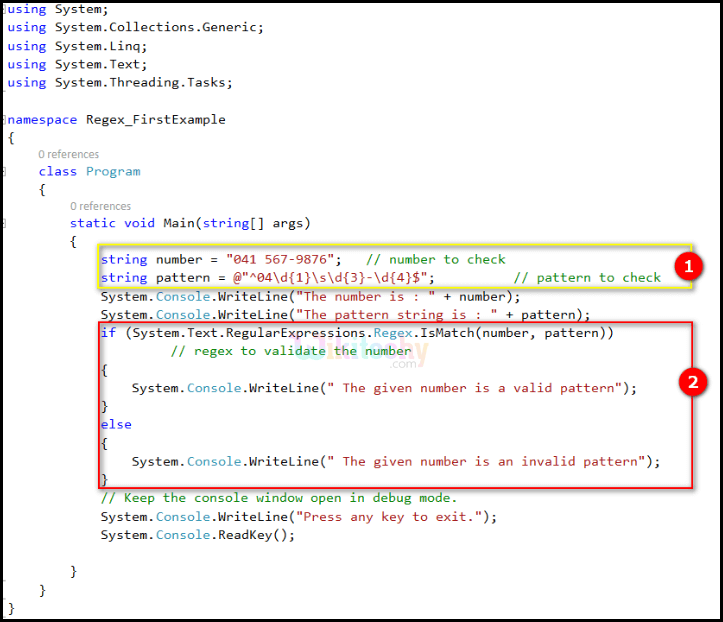The lines of code below declare two string variables, one with a string of numbers and other with a string pattern to match a number pattern as follows:

`string number = "041 567-9876"; // number to checkstring pattern = @"^40\d{1}\s\d{3}-\d{4}\$"; // pattern to check`Now the pattern string is checked against the string of numbers and if the check satisfies, a message is shown at the console as “The given number is a valid pattern” else “The given number is an invalid pattern”. This is done by the following lines of code:

`if (System.Text.RegularExpressions.Regex.IsMatch(number, pattern))// regex to validate the number{	    System.Console.WriteLine(" The given number is a valid pattern");}else{    System.Console.WriteLine(" The given number is an invalid pattern");}`

## Output :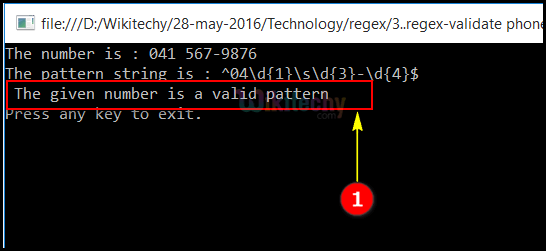The string pattern “^04\d{1}\s\d{3}-\d{4}\$" checks whether the string "041 567-9876" contains the same pattern. Since the check is satisfied here, the output is displayed as “The given number is a valid pattern”.

• Try out with an invalid number which does not start with 04.
`string number = "031 567-9876";  		       // number to checkstring pattern = @"^04\d{1}\s\d{3}-\d{4}\$";          // pattern to check`
• The output is -> The given number is an invalid pattern.

Bug Bounty
Webinar